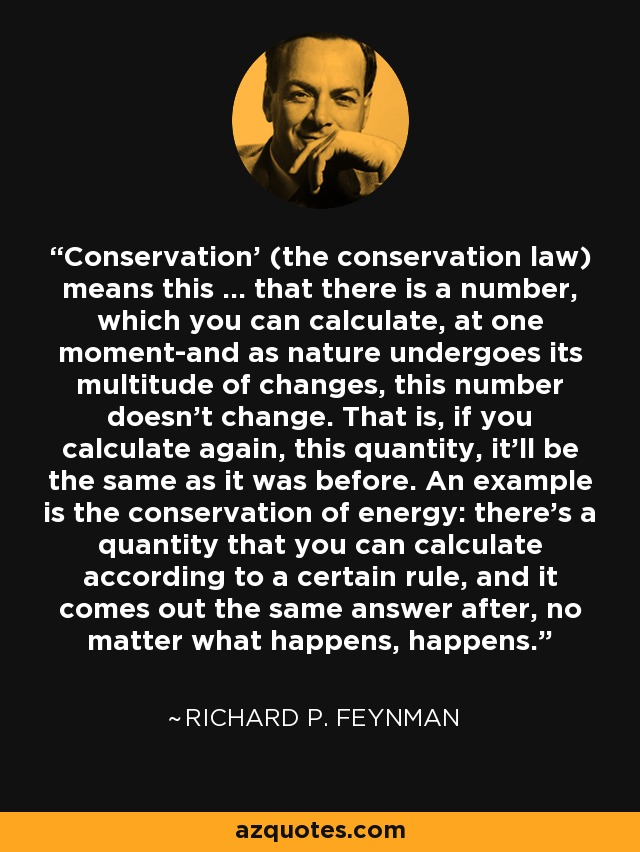Authors:
• 'Conservation' (the conservation law) means this ... that there is a number, which you can calculate, at one moment-and as nature undergoes its multitude of changes, this number doesn't change. That is, if you calculate again, this quantity, it'll be the same as it was before. An example is the conservation of energy: there's a quantity that you can calculate according to a certain rule, and it comes out the same answer after, no matter what happens, happens.

TopicsEmbed: